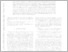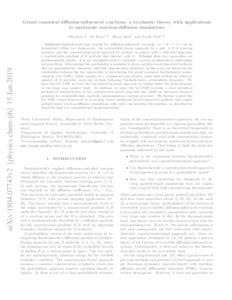Repository: Freie Universität Berlin, Math Department

# Grand canonical diffusion-influenced reactions: a stochastic theory with applications to multiscale reaction-diffusion simulations

del Razo, M.J. and Qian, H. and Noé, F. (2018) Grand canonical diffusion-influenced reactions: a stochastic theory with applications to multiscale reaction-diffusion simulations. J. Chem. Phys., 149 (4). 044102. ISSN 0021-9606, ESSN: 1089-7690Preview

8MB

Official URL: https://dx.doi.org/10.1063/1.5037060

## Abstract

Smoluchowski-type models for diffusion-influenced reactions (A+B -> C) can be formulated within two frameworks: the probabilistic-based approach for a pair A, B of reacting particles and the concentration-based approach for systems in contact with a bath that generates a concentration gradient of B particles that interact with A. Although these two approaches are mathematically similar, it is not straightforward to establish a precise mathematical relationship between them. Determining this relationship is essential to derive particle-based numerical methods that are quantitatively consistent with bulk concentration dynamics. In this work, we determine the relationship between the two approaches by introducing the grand canonical Smoluchowski master equation (GC-SME), which consists of a continuous-time Markov chain that models an arbitrary number of B particles, each one of them following Smoluchowski's probabilistic dynamics. We show that the GC-SME recovers the concentration-based approach by taking either the hydrodynamic or the large copy number limit. In addition, we show that the GC-SME provides a clear statistical mechanical interpretation of the concentration-based approach and yields an emergent chemical potential for nonequilibrium spatially inhomogeneous reaction processes. We further exploit the GC-SME robust framework to accurately derive multiscale/hybrid numerical methods that couple particle-based reaction-diffusion simulations with bulk concentration descriptions, as described in detail through two computational implementations.

Item Type: Article SFB1114-Preprint in arXiv:1804.07743 (https://arxiv.org/abs/1804.07743) Mathematical and Computer Sciences > Mathematics > Applied Mathematics Department of Mathematics and Computer Science > Institute of Mathematics > Comp. Molecular Biology 2290 Silvia Hoemke 06 Feb 2019 15:57 26 Jun 2019 12:39

Repository Staff Only: item control page